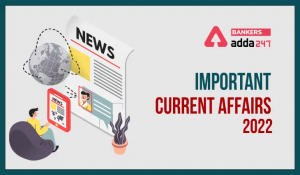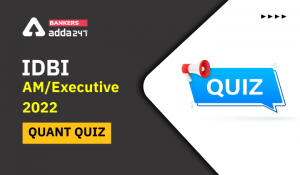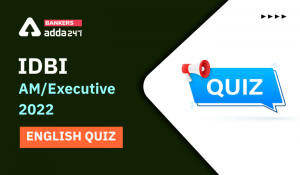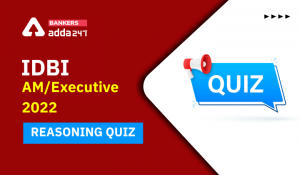Latest Banking jobs   »   Reasoning Ability Quiz For IDBI AM/Executive...

# Reasoning Ability Quiz For IDBI AM/Executive 2022- 6th June

Directions (1-5): Following questions are based on the five three-digit numbers given below:

437   341   669   492   136

Reasoning Quiz For IDBI AM/Executive 2022 6th June CHECK IN HINDI

Q1. If the first and second digits of each number are interchanged, then multiply the second digit of the largest number with third digit of the smallest number.
(a) 36
(b) 24
(c) 20
(d) 32
(e) 28

Q2. If all the digits of every number are added within the number, then which number’s sum of digits will be smallest?
(a) 437
(b) 341
(c) 669
(d) 492
(e) 136

Q3. If the first digit of every number is removed, how many numbers will be divisible by 3?
(a) One
(b) Two
(c) Three
(d) More than three
(e) None

Q4. If the digits of all the numbers are arranged in ascending order within the number from left to right, which will be the third largest number?
(a) 437
(b) 341
(c) 669
(d) 492
(e) 136

Q5. What will be the resultant, if the second digit of the highest number is divided by the second digit of the lowest number?
(a) 3
(b) 2
(c) 1
(d) 5
(e) None of the above

Direction (6-10): Following questions are based on the alphanumeric-symbol series given below:
7 H β 5 % T I M 6 \$ E 8 G 3 S N @ A # C D 4 8 U & D

Q6. If all the numbers, vowels and the symbol ‘&’ is removed from the above arrangement then which of the following will be the eighth element from the left end?
(a) @
(b) G
(c) N
(d) S
(e) None of these

Q7. If the first fifteen elements in the above sequence are written in reverse order then which of the following will be the sixteenth element from the right end?
(a) N
(b) 7
(c) %
(d) T
(e) None of these

Q8. If all the letters are changed to their next letter according to the alphabetical series, then which of the following will be the seventh to the right of the tenth element from the left end?
(a) T
(b) D
(c) B
(d) @
(e) None of these

Q9. How many such numbers are there in the above arrangement each of which is immediately followed by a symbol and also immediately preceded by a letter?
(a) Three
(b) Two
(c) One
(d) Four
(e) None of these

Q10. Four among the following five are same in a certain way according their arrangement in the series and forms a group. Find the one that does not belong to the group?
(a) 5%7
(b) 6\$T
(c) 8G6
(d) SNA
(e) 8UC

Direction (11-15): Following questions are based on the five three-letter words given below:

NLT   DCA   MCU   RBM   GLO

Q11. If all the letters within the words are arranged in reverse alphabetical order, after that arrange the words in alphabetical order from left to right. Which of the following word is 2nd from the right end?
(a) NLT
(b) MCU
(c) DCA
(d) RBM
(e) GLO

Q12. If in each word, first and the last letter interchange their positions with each other, then all the word are arranged in alphabetical order from left to right. Which of the following word is 2nd from the left end?
(a) NLT
(b) MCU
(c) DCA
(d) RBM
(e) GLO

Q13. If in each word, every consonant is changed to the previous letter as per English alphabetical order, then how many words have at least two vowels?
(a) 0
(b) 1
(c) 2
(d) 3
(e) 4

Q14. How many letters are there in English alphabetical series between the 2nd letter of the word which is 2nd from the left end and 2nd letter of the word which is at the right end?
(a) 4
(b) 3
(c) 5
(d) 6
(e) More than 6

Q15. If in each word, consonant changes to the next letter and vowel changes to the previous letter according to the alphabetical order, then how many words have no vowel?
(a) 2
(b) 1
(c) 4
(d) More than 4
(e) 3

SOLUTIONS

S1. Ans. (b)
Sol. Given Series – 437 341 669 492 136
After operation – 347 431 669 942 316
Second digit of the largest number – 4 (942)
Third digit of the smallest number – 6 (316)
So, required multiplication – 4 x 6 – 24

S2. Ans. (b)
Sol. Given Series – 437 341 669 492 136
Sum – 14 8 21 15 10
After adding all the digits of every number, we find that 341’s sum is be smallest.

S3. Ans. (b)
Sol. Given Series – 437 341 669 492 136
After removing first number – 37 41 69 92 36
So, there are two numbers (69, 36) which are divisible by 3.

S4. Ans. (d)
Sol. Given Series – 437 341 669 492 136
After operation – 347 134 669 249 136
So, third largest number – 492

S5. Ans. (b)
Sol. Given Series – 437 341 669 492 136
Second digit of the highest number – 6
Second digit of the lowest number – 3
So, required division – 2

S6. Ans. (d)
Sol. Given Series – 7 H β 5 % T I M 6 \$ E 8 G 3 S N @ A # C D 4 8 U & D
After dropping – H β % T M \$ G S N @ # C D D
Therefore, option (d) is correct.

S7. Ans. (c)
Sol. Given Series – 7 H β 5 % T I M 6 \$ E 8 G 3 S N @ A # C D 4 8 U & D
Required reversal – S 3 G 8 E \$ 6 M I T % 5 β H 7 N @ A # C D 4 8 U & D
Therefore, option (c) is the correct answer.

S8. Ans. (d)
Sol. Given Series – 7 H β 5 % T I M 6 \$ E 8 G 3 S N @ A # C D 4 8 U & D
After changing – 7 I β 5 % U J N 6 \$ F 8 H 3 T O @ B # D E 4 8 V & E
Therefore, option (d) is the correct answer.

S9. Ans. (c)
Sol. Given Series – 7 H β 5 % T I M 6 \$ E 8 G 3 S N @ A # C D 4 8 U & D
Therefore, option (c) is the correct answer.

S10. Ans. (d)
Sol. Given Series – 7 H β 5 % T I M 6 \$ E 8 G 3 S N @ A # C D 4 8 U & D
Therefore, option (d) is the correct answer.

S11. Ans. (a)
Sol. After all letters in reverse order -TNL DCA UMC RMB OLG
After all word in alphabetical order -DCA OLG RMB TNL UMC
So, the word is TNL which is formed from NLT.

S12. Ans. (d)
Sol. After interchanging the letter – TLN ACD UCM MBR OLG
After all word in alphabetical order- ACD MBR OLG TLN UCM
So, 2nd from the left end – MBR which is formed from RBM.

S13. Ans. (a)
Sol. Given Series – NLT DCA MCU RBM GLO
After changing – MKS CBA LBU QAL FKO
Hence, no word has at least two vowels.

S14. Ans. (e)
Sol. Given Series – NLT DCA MCU RBM GLO
Second letter of the second word from the left end – C
Second letter of the word which is right end – L
So, there are 8 letters between C and L.

S15. Ans. (e)
Sol. Given Series – NLT DCA MCU RBM GLO
After changing – OMU EDZ NDT SCN HMN
Hence, there are three words.

#### Congratulations!Incorrect details? Fill the form again here

•Reasoning Ability Quiz For IBPS RRB PO P...
•Important Current Affairs Quiz for Bank ...
•Quantitative Aptitude Quiz For IDBI AM/E...
•English Quizzes For IDBI AM/Executive 20...
•Reasoning Ability Quiz For IBPS RRB PO P...
•Reasoning Ability Quiz For IDBI AM/Execu...